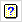1. Home
2. Multi-treatment Meta Analysis of Summaries Options

# Multi-treatment Meta Analysis of Summaries Options

Use this to select the model for a multi-treatment meta analysis of summaries and the output and graphs to be generated from this.## Display

This specifies which items of output are to be produced by the analysis.

 Model Description of the model fitted by the analysis Wald tests Wald tests for fixed model terms and accompanying F-statistics Variance components Estimated variance components and parameters Treatment means Predicted treatment means averaged over the experiments Treatment effects Estimates of treatment effects averaged over the experiments Variance-covariance matrix Variance-covariance matrix for the variance parameters Deviance The residual deviance Covariance model Estimated between-experiment variance-covariance model in matrix format Monitoring Monitoring information at each iteration Residual plot Uses the VPLOT procedure to produce residual diagnostic plots from the REML analysis. The type of residuals plotted is controlled by the Method for residuals setting Residual checks Uses the VCHECK procedure to check the residuals for outliers and variance stability

## Model

This specifies the between-experiment variance-covariance model for the meta analysis. The first two models fit the experiment term as fixed effect, and the others as a random effect.

 Identity A common variance for all experiments and no correlations between experiments Diagonal matrix (heteroscedastic) A separate variance for each experiment and no correlations between experiments Compound symmetry A common variance for all experiments with a common covariance between experiments Heterogeneous compound symmetry A separate variance for each experiment with a common correlation between experiments Unstructured A separate variance for each experiment and separate covariances between experiments First order analytic model with common variance A common variance for all experiments and structured covariances between experiments using a factor analytic model with one term Second order analytic model with common variance A common variance for all experiments and structured covariances between experiments using a factor analytic model with two terms First order analytic model A separate variance for each experiment and structured covariances between experiments using a factor analytic model with one term

See the VMETA procedure for more information on the structure of each model.

## Standard errors

Tables of means and effects can be accompanied by estimates of standard errors if selected above. You can choose whether Genstat computes standard errors or standard errors of differences (SEDs) for the tables giving all values or just a summary of these.

 Differences Display the minimum, mean and maximum standard errors of differences between means or effects. Estimates Display the minimum, mean and maximum standard errors of estimates for means or effects. All differences Display all the standard errors of differences between pairs of means or effects. All estimates Display all the standard errors of estimates for means or effects.

## Maximum iterations

This specifies the maximum number of iterations to use to optimize the REML likelihood.

## Method for residuals

The list allows selection of the type of residuals to be plotted in the Residual plot. This is only enabled if a residual plot has been selected.

 Combine all random terms Use the residuals combined from all random terms. Final random term only Use the residuals from the final random term. Standardized residuals from all random terms Use the standardized residuals after combining them from all random terms. Standardized residuals from final random term only Use the standardized residuals from the final random term.

## Action buttons

 OK Save the settings and close the dialog. Cancel Close the dialog without further changes. Defaults Reset the options to the initial settings when the dialog was first opened.Clear all check boxes and fields.Help about this menu.